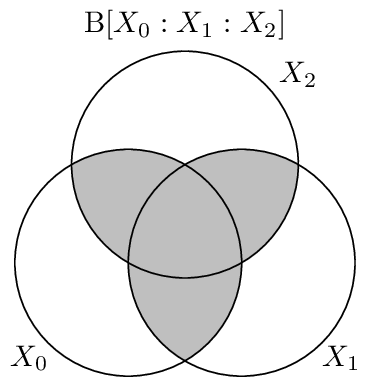# Dual Total Correlation¶

The dual total correlation [Han75], or binding information [AP12], is yet another generalization of the Mutual Information. It is the amount of information that is shared among the variables. It is defined as:

$\begin{split}\B{X_{0:n}} &= \H{X_{0:n}} - \sum \H{X_i | X_{\{0..n\}/i}} \\ &= - \sum_{x_{0:n} \in X_{0:n}} p(x_{0:n}) \log_2 \frac{p(x_{0:n})}{\prod p(x_i|x_{\{0:n\}/i})}\end{split}$

In a sense the binding information captures the same information that the Total Correlation does, in that both measures are zero or non-zero together. However, the two measures take on very different quantitative values for different distributions. By way of example, the type of distribution that maximizes the total correlation is a “giant bit”:

In : In : from dit.multivariate import binding_information, total_correlation


For the same distribution, the dual total correlation takes on a relatively low value. On the other hand, the type of distribution that maximizes the dual total correlation is a “parity” distribution:

In : In : from dit.example_dists import n_mod_m


## Relationship to Other Measures¶

The dual total correlation obeys particular bounds related to both the Entropy and the Total Correlation:

$\begin{split}0 \leq & \B{X_{0:n}} \leq \H{X_{0:n}} \\ \frac{\T{X_{0:n}}}{n-1} \leq & \B{X_{0:n}} \leq (n-1)\T{X_{0:n}}\end{split}$

## Visualization¶

The binding information, as seen below, consists equally of the information shared among the variables.## API¶

dual_total_correlation(dist, rvs=None, crvs=None, rv_mode=None)[source]

Calculates the dual total correlation, also known as the binding information.

Parameters
• dist (Distribution) – The distribution from which the dual total correlation is calculated.

• rvs (list, None) – The indexes of the random variable used to calculate the dual total correlation. If None, then the dual total correlation is calculated over all random variables.

• crvs (list, None) – The indexes of the random variables to condition on. If None, then no variables are condition on.

• rv_mode (str, None) – Specifies how to interpret rvs and crvs. Valid options are: {‘indices’, ‘names’}. If equal to ‘indices’, then the elements of crvs and rvs are interpreted as random variable indices. If equal to ‘names’, the the elements are interpreted as random variable names. If None, then the value of dist._rv_mode is consulted, which defaults to ‘indices’.

Returns

B – The dual total correlation.

Return type

float

Raises

ditException – Raised if dist is not a joint distribution or if rvs or crvs contain non-existant random variables.